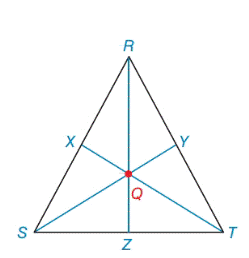Chapter 7.2, Problem 28E### Elementary Geometry for College St...

6th Edition
Daniel C. Alexander + 1 other
ISBN: 9781285195698

#### Solutions

Chapter
Section### Elementary Geometry for College St...

6th Edition
Daniel C. Alexander + 1 other
ISBN: 9781285195698
Textbook Problem
1 views

# Given: Isosceles Δ R S T R S = R T = 10         a n d         S T = 16. Medians R Z ¯ , T X ¯ and S Y ¯ meet at centroid Q .Find: R Q         and           Q TExercises 29, 30

To determine

To find:

The RQandQT in the given isosceles triangle RST.

Explanation

Definition:

The three medians of a triangle are congruent at a point that is two-thirds the distance from any vertex to the midpoint of the opposite side.

Formula used:

The triangle RST, with sides a,bandc opposite vertices R,SandT. Then the length of the median ma form R is given by

ma=122(b2+c2)a2

The length of the median mc form T is given by

mc=122(a2+b2)c2

Where ma,mc denotes the length of the median

Given:

In ΔRST, medians RZ¯, TX¯ and SY¯ intersect at centroid Q. If RS=RT=10andST=16.

Calculation:

The point of concurrence Q for the three medians of a triangle is known as centroid of the triangle. In ΔMNP, medians MB¯, NA¯ and PC¯ intersect at centroid Q.

According to the definition,

RQ=23(RZ)(1)QT=23(TX)(2)

Given RS=RT=10andST=16.

The triangle RST, with sides a,bandc opposite vertices R,SandT.

So, ST=16=a,RT=10=bandRS=10=c.

We sketch the figure of the isosceles triangle RST with sides a,bandc

First we need to find length of the median RZ,

Using the above formula,

The length of the median ma form R is given by

RZ=ma=122(b2+c2)a2=122(102+102)162=122(100+100)256=122(200)256=12400256=12144Ȁ

### Still sussing out bartleby?

Check out a sample textbook solution.

See a sample solution

#### The Solution to Your Study Problems

Bartleby provides explanations to thousands of textbook problems written by our experts, many with advanced degrees!

Get Started

#### 21. If has the graph in Problem 5(a), find the following. (a) f(9) (b) f(5)

Mathematical Applications for the Management, Life, and Social Sciences

#### In Exercises 3540, rationalize the numerator of each expression. 39. 1+x+2x+2

Applied Calculus for the Managerial, Life, and Social Sciences: A Brief Approach

#### Simplify the expressions in Exercises 97106. 21/32122/321/3

Finite Mathematics and Applied Calculus (MindTap Course List)